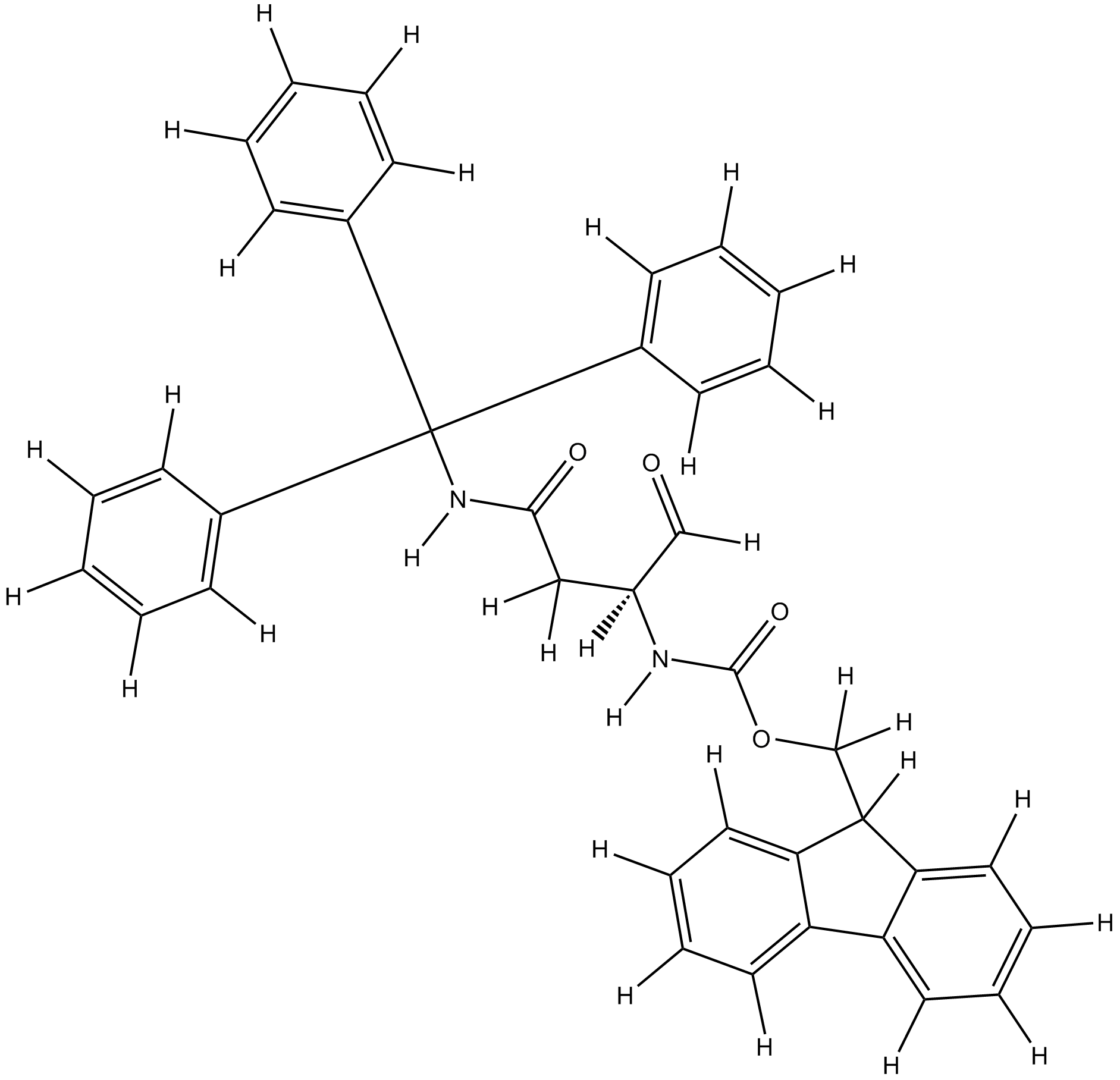Close
• Setting

# Fmoc-Asn(Trt)-Wang resin

Catalog No.
A6845Grouped product items
SizePriceStock Qty
5g
\$70.00
Ship with 10-15 days
25g
\$279.00
Ship with 20-30 days

Tel: +1-832-696-8203

Email: [email protected]

Worldwide Distributors

## Background

Fmoc-Asn(Trt)-Wang resin

## Chemical Properties

 Storage Store at 4°C M.Wt 0.3-0.8mmol/g Cas No. N/A Formula 100-200:mesh,1%DVB Chemical Name (S)-(9H-fluoren-9-yl)methyl (1,4-dioxo-4-(tritylamino)butan-2-yl)carbamate SDF Download SDF Canonical SMILES O=C(C([H])([H])[[email protected]](C([H])=O)([H])N([H])C(OC([H])([H])C1([H])C2=C([H])C([H])=C([H])C([H])=C2C3=C([H])C([H])=C([H])C([H])=C13)=O)N([H])C(C4=C([H])C([H])=C([H])C([H])=C4[H])(C5=C([H])C([H])=C([H])C([H])=C5[H])C6=C([H])C([H])=C([H])C([H])=C6[H] Shipping Condition Ship with blue ice, or upon other requests. General tips For obtaining a higher solubility, please warm the tube at 37°C and shake it in the ultrasonic bath for a while. We do not recommend long-term storage for the solution, please use it up soon.

## Quality Control

### Quality Control & MSDS

View current batch:

### Chemical structure